# Chapter 8 Minitab Statistical decision making Confidence intervals

• Slides: 16Chapter 8 Minitab: Statistical decision making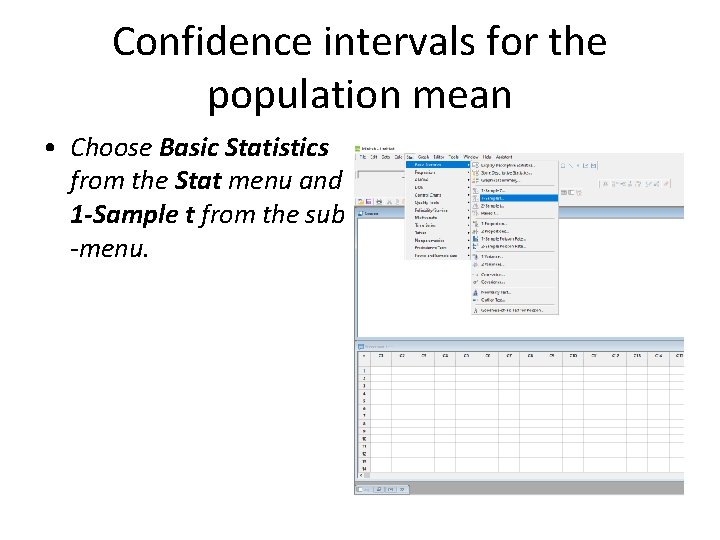Confidence intervals for the population mean • Choose Basic Statistics from the Stat menu and 1 -Sample t from the sub -menu.• In the top right of the new window select Summarized data.• For Example 8. 6 enter 40 as the Sample size, 3. 47 as the Mean, and 0. 82 as the Standard deviation. • Click Options.• In the Options window type 90. 0 in the space to the right of Confidence level. • Click OK then OK in the One-Sample t for the Mean window.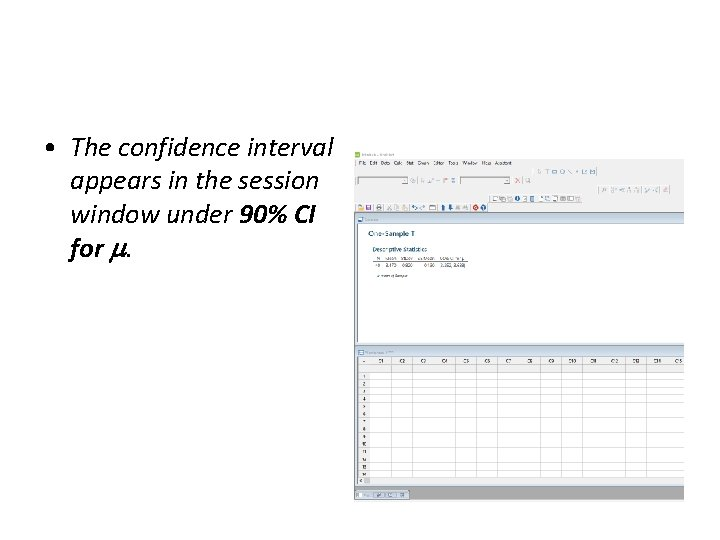• The confidence interval appears in the session window under 90% CI for .Hypothesis tests for differences between population means • Select Basic Statistics from the Stat menu then 2 -Sample t from the sub-menu.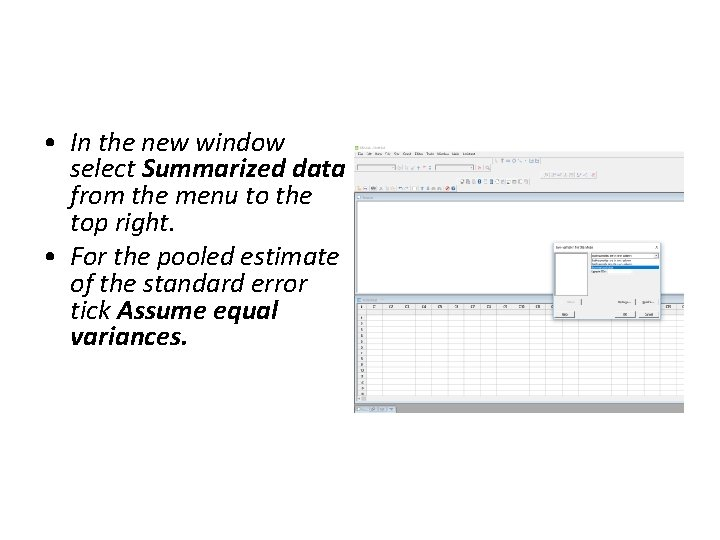• In the new window select Summarized data from the menu to the top right. • For the pooled estimate of the standard error tick Assume equal variances.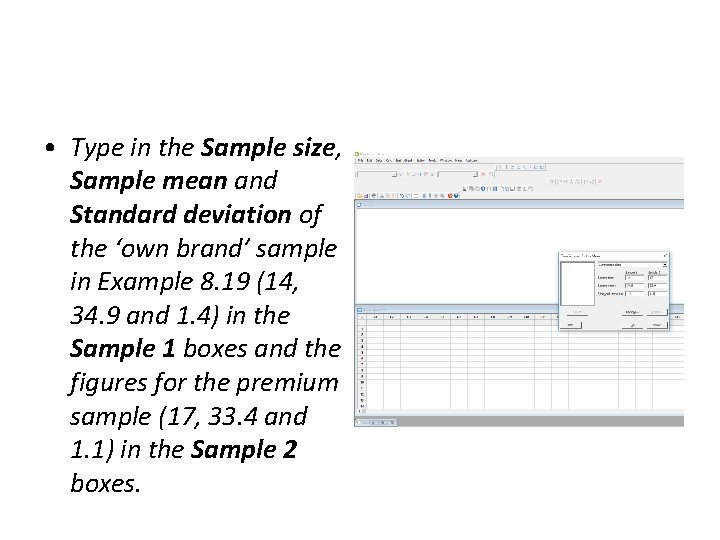• Type in the Sample size, Sample mean and Standard deviation of the ‘own brand’ sample in Example 8. 19 (14, 34. 9 and 1. 4) in the Sample 1 boxes and the figures for the premium sample (17, 33. 4 and 1. 1) in the Sample 2 boxes.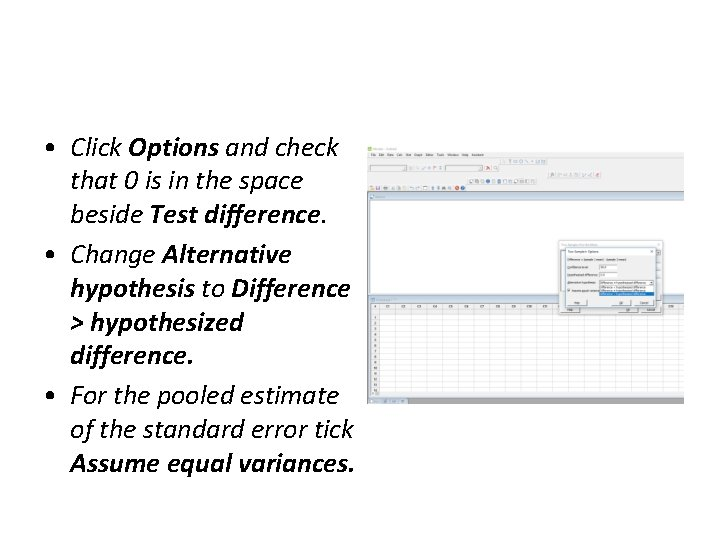• Click Options and check that 0 is in the space beside Test difference. • Change Alternative hypothesis to Difference > hypothesized difference. • For the pooled estimate of the standard error tick Assume equal variances.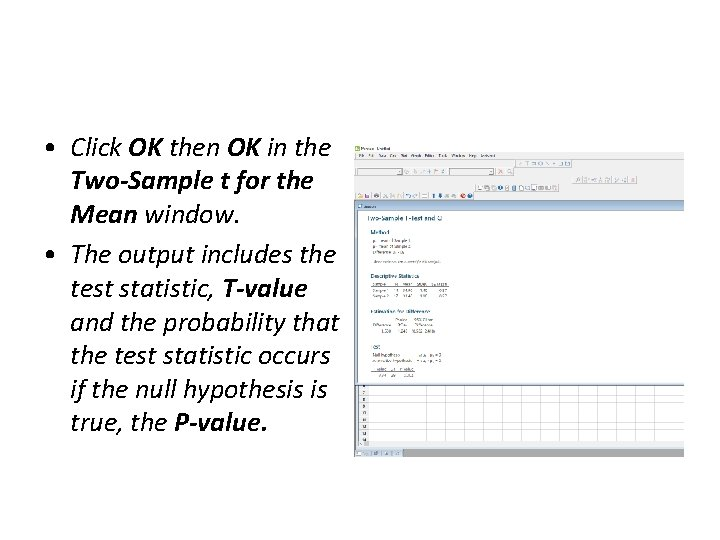• Click OK then OK in the Two-Sample t for the Mean window. • The output includes the test statistic, T-value and the probability that the test statistic occurs if the null hypothesis is true, the P-value.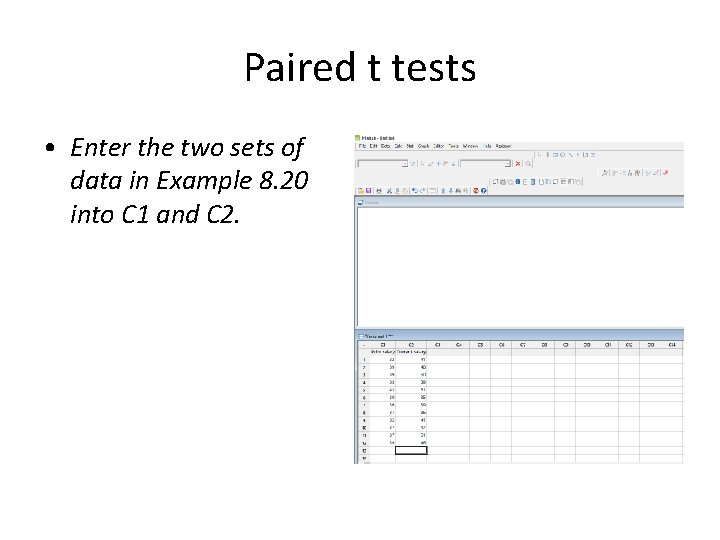Paired t tests • Enter the two sets of data in Example 8. 20 into C 1 and C 2.• Choose Basic Statistics from the Stat menu then Paired t from the sub-menu.• Check that Each sample is in a column is selected. • Type C 2 for the location of Sample 1 and C 1 for the location of Sample 2.• Click Options, type 8 as the Hypothesized difference and change the Alternative hypothesis to Difference < hypothesized difference. • Click OK then OK in the Paired t for the Mean window.• The output in the session window includes the test statistic, Tvalue and the probability that the test statistic occurs if the null hypothesis is true, P -value.